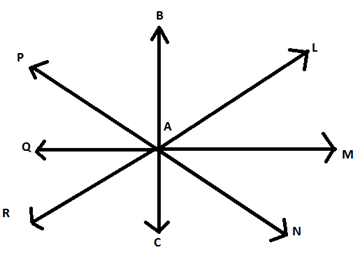RS Aggarwal Class 6 Solutions Chapter 11 - Line Segment Ray And Line

RS Aggarwal Class 6 Chapter 11 - Line Segment Ray And Line Solutions Free PDF

In this chapter, the students will learn basic geometric concepts like what is a line segment, how to draw a line segment. Along with this students will the difference between a line segment, line, and ray. To score good marks and to answer all the questions in examination students are advised to practice questions from RS Aggarwal for class 6. To help students solve all the RS Aggarwal questions we have provided the RS Aggarwal class 6 solutions chapter 11 line segment, ray, and line.

Exercise 11A

1. Name all the line segments in each of the following figures: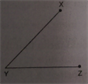(i)

The line segments are:

$\overline{YX}$

This is because it has two end point Y and X.

$\overline{YZ}$

This is because it has two end point Y and Z.

(ii)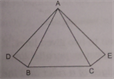$\overline{AD}$

This is because it has two end point A and D.

$\overline{AB}$

This is because it has two end point A and B.

$\overline{AC}$

This is because it has two end point A and C.

$\overline{AE}$

This is because it has two end point A and E.

$\overline{DB}$

This is because it has two end point D and B.

$\overline{BC}$

This is because it has two end point B and C.

$\overline {CE}$

This is because it has two end point C and E.

(iii)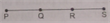(i) $\overline {PS}$

This is because it has two end point P and S.

$\overline {PQ}$

This is because it has two end point P and Q.

$\overline {QR}$

This is because it has two end point Q and R.

$\overline {RS}$

This is because it has two end point R and S.

$\overline {PR}$

This is because it has two end point P and R.

$\overline {QS}$

This is because it has two end point Q and S.

2. Identify and name the line segments and rays in each of the following figures:

(i)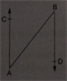(i) Line segment is $\overline{AB}$. This is because it has two end point A and B.

Rays are:

$\overrightarrow {AC}$

This is because it has only one end point A.

$\overrightarrow {BD}$

This is because it has only one end point B.

(ii)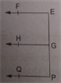Line segments are:

$\overline{EP}$

This is because it has two end point E and P.

$\overline{EG}$

This is because it has two end point E and G.

$\overline {GP}$

This is because it has two end point G and P.

Rays are:

$\overrightarrow {EF}$

This is because it has only one end point E.

$\overrightarrow {GH}$

This is because it has only one end point G.

$\overrightarrow {PQ}$

This is because it has only one end point P.

(iii)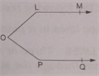Line segments are:

$\overline {OL}$

This is because it has two end point O and L.

$\overline {OP}$

This is because it has two end point O and P.

Rays are:

$\overrightarrow {LM}$

This is because it has only one end point L.

$\overrightarrow {PQ}$

This is because it has only one end point P.

3. In the adjoining figure, name

(i) four line segments;

(ii) four rays;

(iii) two non-intersecting line segments.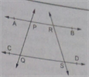Line segments are:

(i) $\overline {PR}$

This is because it has two end point P and R.

$\overline {QS}$

This is because it has two end point Q and S.

$\overline {PQ}$

This is because it has two end point P and Q.

$\overline {RS}$

This is because it has two end point R and S.

(ii) Rays are:

$\overrightarrow {PA}$

This is because it has only one end point P.

$\overrightarrow {RB}$

This is because it has only one end point R.

$\overrightarrow {QC}$

This is because it has only one end point Q.

$\overrightarrow {SD}$

This is because it has only one end point S.

(iii) $\overline {PR}$ and $\overline {QS}$ are the two non-intersecting line segments as they do not have any point in common.

4. What do you mean by collinear points?

(i) How many lines can you draw passing through three collinear points?

(ii) Given three collinear points A, B, C, how many line segments do they determine? Name them.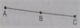COLLINEAR POINTS:

Three or more points in a plane are said to be collinear if they all lie in the same line. This line is called the line of collinearity for the given points.

(i) We can draw only one line passing through three collinear points.

(ii) 3 Line segments are:

$\overline {AB}$

This is because it has two end point A and B.

$\overline {BC}$

This is because it has two end point B and C.

$\overline {AC}$

This is because it has two end point A and C.

5. In the adjoining figure, name:

(i) four pairs of intersecting lines

(ii) four collinear points

(iii) three non-collinear points

(iv) three concurrent lines

(v) three lines whose point of intersection is P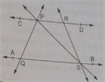(i) PS and AB intersecting at S.

CD and RS intersecting at R.

PS and CD intersecting at P.

AB and RS intersecting at S.

(ii) A, Q, S and B are four collinear points as they all lie on the same line AB.

(iii) A, C and B are non-collinear points as they do not lie on the same line.

(iv) PS, RS and AB are three concurrent lines passing through the same point S.

(v) PS, PQ and CD have common point of intersecting P.

6. Mark three non-collinear points A, B, C, as shown. Draw lines through these points taking two at a time. Name the lines. How much such different lines can be drawn?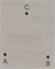Taking point A and B, we can draw only one line AB.

Taking point B and C, we can draw only one line BC.

Taking point A and C, we can draw only one line AC.

We can draw only three lines through these non-collinear points A, B and C.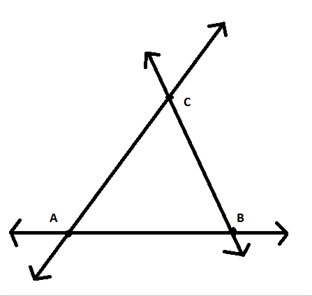7. Count the number of line segments drawn in each of the following figures and name them.

(i)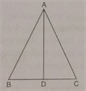There are 6 line segments. These are:

$\overline {AB}$ (with end point A and B)

$\overline {AC}$ (with end point A and C)

$\overline {AD}$(with end point A and D)

$\overline {BC}$ (with end point B and C)

$\overline {BD}$ (with end point B and D)

$\overline {CD}$ (with end point C and D)

(ii)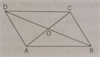$\overline {AB}$ (with end point A and B)

$\overline {BC}$ (with end point B and C)

$\overline {CD}$ (with end point C and D)

$\overline {AD}$ (with end point A and D)

$\overline {AC}$ (with end point A and C)

$\overline {BD}$ (with end point B and D)

$\overline {AO}$ (with end point A and O)

$\overline {CO}$ (with end point C and O)

$\overline {BO}$ (with end point B and O)

$\overline {DO}$ (with end point D and O)

(iii)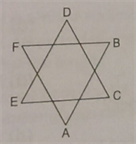There are 6 line segments, they are:

$\overline {AB}$

$\overline {AF}$

$\overline {FB}$

$\overline {EC}$

$\overline {ED}$

$\overline {DC}$

(iv)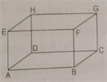There are 12 line segments, they are:

$\overline {AB}$

$\overline {AD}$

$\overline {AE}$

$\overline {BC}$

$\overline {BF}$

$\overline {CG}$

$\overline {CD}$

$\overline {HG}$

$\overline {HE}$

$\overline {DH}$

$\overline {EF}$

$\overline {GF}$

8. Consider the line PQ given below and find whether the given statements are true and false: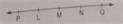(i) M is a point on ray $\overrightarrow {NQ}$.

M is outside ray NQ.

(ii) L is a point on ray $\overrightarrow {MP}$.

L is placed between M and P.

(iii) Ray $\overrightarrow {MQ}$ is different from ray $\overrightarrow {NQ}$.

Ray MQ is extended endlessly from M to Q and ray NQ is extended endlessly from N to Q.

(iv) L, M, N are points on line segment $\overline {LN}$.

(v) Ray $\overrightarrow {LP}$ is different from ray $\overrightarrow {LQ}$.

$\overrightarrow {LP}$ is extended from L to P.

$\overrightarrow {LQ}$is extended from L to Q.

9. Write ‘T’ for true and ‘F’ for false in case of each of the following statements:

(i) Every point has a size. – (False)

A point does not have any length, breadth or thickness.

(ii) A line segment has no length. – (False)

A line segment has a definite length.

(iii) Every ray has a finite length. – (False)

A ray has no definite length.

(iv) The ray $\overrightarrow {AB}$ is the same as the ray $\overrightarrow {BA}$. – (False)

Ray AB has initial point A and is extended endlessly towards B, while ray BA has initial point B and is extended endlessly towards A.

(v) The line segment $\overline {AB}$is the same as the line segment $\overline {BA}$ – (True)

This is because both the line segments have definite length with end point A and B.

(vi) The line AB is the same as the line BA. – (True)

This is because it neither has a definite length nor any end point.

(vii) Two points A and B in a plane determine a unique line segment. – (True)

Only one line segment can pass through the two given points.

(viii) Two intersecting lines intersect at a point. – (True)

(ix) Two intersecting planes intersect at a point. – (False)

Two intersecting planes intersect at a line.

(x) If point A, B, C are collinear and point C, D, E are collinear the A, B, C, D, E are collinear. – (False)

Different set of collinear points need not be collinear.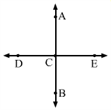(xi) One and only one ray can be drawn with a given end point. – (False)

With point P, endless rays (like PA, PB, PC, PD, PE, PF) can be drawn.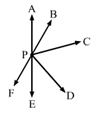(xii) One and only one line can be drawn to pass through two given points. – (True)

Two points define on unique line.

(xiii) An unlimited number of lines can be drawn to pass through a given points. – (True)

10. Fill in the blanks:

(i)A line segment has a definite length.

(ii) A ray has oneend point.

(iii) A line has no end point.

(iv) A ray has no definite length.

(v) A line cannot be drawn on a paper.

Exercise 11B

OBJECTIVE QUESTIONS

Mark correct against the correct answer in each of the following:

1. Which of the following has no. end points?

(a) A line segment (b) A ray (c) A line (d) none of these

(c) A line does not have any end point. It is a line segment that is extended endlessly on both sides.

2. Which of the following has one end point?

(a) A line (b) A ray (c) A line segment (d) none of these

(b) A ray has one end point, which is called an initial point. It is extended endlessly towards the other direction.

3. Which of the following has two end points?

(a) A line segment (b) A ray (c) A line (d) none of these

(a) A line segment has two end points and a definite length that can be measured.

4. Which of the following has a definite length?

(a) A line (b) A line segment (c) A ray (d) none of these

(b) A line segment has a definite length that can be measured by the ruler and, therefore it can be drawn on a paper.

5. Which of the following can be drawn on a piece of paper?

(a) A line (b) A line segment (c) A ray (d) A plane

(b) A line segment has a definite length that can be measured by a ruler. So it can be drawn on a paper.

6. How many lines can be drawn passing through a given point?

(a) one only (b) two (c) three (d) unlimited number

(d) Unlimited number of lines can be drawn.

7. How many lines can be drawn passing through two given points?

(a) one only (b) two (c) three (d) unlimited number

(a) only one line can be drawn that passes through two given points.

8. Two planes intersect

(a) at a point (b) in a plane (c) in a line (d) none of these

(c) Two intersecting planes intersect in a line.

9. Two lines intersect

(a) at a point (b) at two points (c) at an infinite number of points (d) in a line

(a) Two lines intersect at a point.

10. Two points in a plane determine.

(a) exactly one line segment (b) exactly two line segment (c) an infinite number of line segments (d) no one of these

(a) exactly one line segment

Two points in a plane determine exactly one line segment with those two points as its end points.

11. The minimum number of points of intersection of three lines in a plane is

(a) 1 (b) 2 (c) 3 (d) 0

(d) 0

Three lines will not necessarily intersect in a plane. Thus, the minimum point of intersection will be 0.

12. The maximum number of points of intersection of three lines in a plane is

(a) 0 (b) 1 (c) 2 (d) 3

(d) 3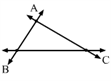The maximum number of points of intersection of three lines that intersect in a plane are three.

13. Choose the correct statement:

(a) every line has a definite length

(b) every ray has a definite length

(c) every line segment has a definite length

(d) none of these

(c) every line segment has a definite length

Every line segment has a definite length, which can be measured using a ruler.

14. Choose a false statement:

(a) Line AB is the same as line BA.

(b) Ray $\overrightarrow {AB}$ is the same as ray $\overrightarrow {BA}$

(c) Line segment $\overline{AB}$ is the same as the line segment $\overline {BA}$

(d) none of these

(b) Ray $\overrightarrow {AB}$ is the same as ray $\overrightarrow {BA}$

This is because the initial points in these rays are A and B, respectively, and are extended endlessly towards B and A, respectively.

15. How many rays can be drawn with a given point as the initial points?

(a) one (d) two (c) an unlimited number (d) a limited number only